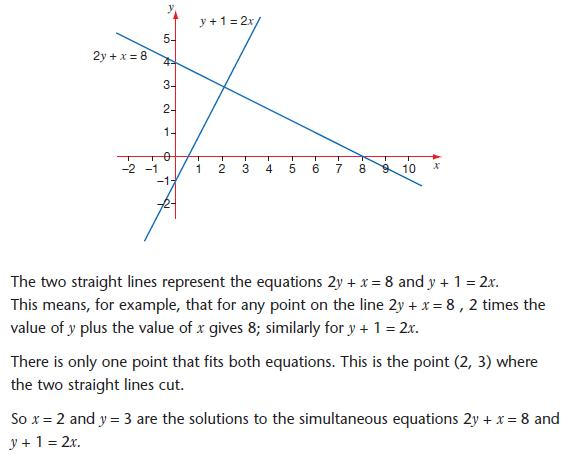## Pre-Algebra Tutorial

#### Intro

This particular tutorial will demonstrate how one can find multiple linear equations. If you believe you need a better understanding of single linear equations, please view my 3 part tutorial (Graph proportional relationship). We will first use an example before graphing. You will be given 2 equations: 1) x+2y=1 2) 2x+4y=2

Before we begin, I must note that the number in front of a variable such as x or y is known as the coefficient. In equation 1, y has a coefficient of 2. In equation 2, x has a coefficient of 2 and y has a coefficient of 4.

Example 1: Confirm the equations
Use the first equation…
x+2y=1 We must first get x by itself by subtracting 2y from each side…
x=1-2y Now we know what x equals!
To solve the actual equation, we have to substitute this into the second equation…
2x+4y=2 Now substitute…
2(1-2y)+4y=2 And now we confirm if the equation is true…
2-4y+4y=2
2=2 True

Now, there are some other tools we can use to help solve different equations-
Example 2: Solve for x
Equation 1) 3x-4y=10 Equation 2) 2x-4y=4
We can actually take the second equation and subtract from the first to eliminate y:
3x-2x-4y-(-4y)=10-4
Remember to put like terms next to each other to make the equation cleaner:
x=6
Note: -4y-(-4y) is the same as -4y+4y, therefore they will eliminate each other

Sometimes there are equations that aren’t quite as simple-
Example 3: Solve for y
Equation 1) 2x+4y=22 Equation 2) 4x+y=16
Since neither of the equations have variables with the same coefficient, we can actually use multiplication to manipulate the equation in a more useful format:
2(2x+4y)=(22)2 Multiply each side by 2 to get…
4x+8y=44 Now each equation has 4x and we can subtract equation 2 from equation 1…
4x-4x+8y-y=44-16 Notice we are eliminating x and solving for y…
7y=28 Now simplify
y=4

To review, example 1 is solving through substitution and examples 2 and 3 use elimination. They are both useful depending on the situation and your preference.

Example 4: Solve for both x and y
Equation 1) 2x-3y=12 Equation 2) 3x+4y=1
Notice that only multiplying one equation will not work. Therefore we will multiply equation 1 by 3 and then multiply equation 2 by 2…
Step 1: 3(2x-3y)=3(12) –> 6x-9y=36 new equation 1
Step 2: 2(3x+4y)=2(1) –> 6x+8y=2 new equation 2
Subtract equation 2 from equation 1 to eliminate x and solve for y
Step 3: 6x-6x-9y-8y=36-2 –> -17y=34 –> y=-2
We have found the value for y. Take this value and use it in any equation to solve for x…
Step 4: 2x-3y=12 –> 2x-3(-2)=12 –> 2x+6=12 –> 2x=6 –> x=3
Not done yet! We have to confirm our answers by substituting in both the values we have found for x and y into one of the equations…
Step 5: 2x-3y=12 –> 2(3)-3(-2)=12 –> 6+6=12 –> 12=12 TRUE

Finally, we can use this visual to see what these 2 equations really representWhen we find the x and y for the equations, we are actually finding the point at which they intersect. From there, we can substitute any coordinates in to find slope, y-intercepts, or confirm if a point is on the line.
Slope of 2y+x=8 using coordinates (2,3) and (8,0)
m=(0-3)/(8-2) –> m=(-3)/6 –> m=-2

#### Sample Problem

1. Solve for x and y using your preferred method and confirm it

2. Find the slope of each equation

Equation 1) 3y+7x=45
Equation 2) y=x-5

#### Solution

1. Step 1: Notice that equation 2 is in a great format and can be placed directly into equation 1
3y+7x=45 –> 3(x-5)+7x=45 –> 3x-15+7x=45 –> 10x+60 –> x=6
Step 2: Now that we know x, lets find y…
y=x-5 –> y=(6)-5 –> y=1
Step 3: Confirm by substituting
3y+7x=45 –> 3(1)+7(6)=45 –> 3+42=45 –> 45=45 TRUE
y=x-5 –> (1)=(6)-5 –> 1=1 TRUE

2. Step 1: We need to find other coordinates for each equation to use in the slope formula
Equation 1) 3y+7x=45 –> Lets use x=0 –> 3y+7(0)=45 –> y=15
Use the points (6,1) and (0,15) to find the slope
m=(15-1)/(0-6) –> m=14/-6 –> m=-7/3

Equation 2) y=x-5 –> lets use x=5 –> y=(5)-5 –> y=0
Use the points (6,1) and (5,0) to find the slope
m=(0-1)/(5-6) –> m=-1/-1 –> m=1

We can confirm that one line is positive slope and the other is negative slope. We can also confirm that they intersect at (6,1).

# About The Author

 Zachary P Math, Financing, And Accounting I am a recent graduate from Kent State University with an Associate of Applied Business and a Bachelor of Business Administration. During my studies, I excelled at math, financing, and accounting and was offered tutoring jobs at the university. Unfortunately, I had to decline the jobs at the time du...
6 SubjectsKnowRo Tutor
8 Tutorials
Ashtabula, OH
Get Tutoring Info

^